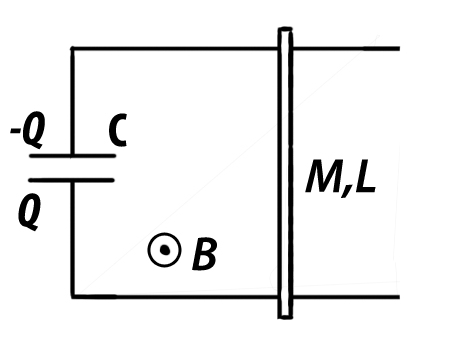# Why are you in a hurry?An arrangement of smooth pair of long conducting rails which are non resisting are joined to a charged capacitor. The rails are connected by a rod of mass $M$, length $L$, and Resistance $R$. A vertical Magnetic Field $B$ is established in the region. The rod starts moving due to the influence of the Magnetic Field.

What should be the mass of the rod $M$ (in mg) so that its Kinetic Energy in steady state is maximum?

Details and Assumptions:
$\bullet L=1m$
$\bullet B=2T$
$\bullet C=1\mu F$
$\bullet R=1$ohm
$\bullet$ The Rod is initially at rest
$\bullet$ The Magnetic Field is constant and uniform in nature

×